MORE IN Finite Element Analysis
MU Mechanical Engineering (Semester 8)
Finite Element Analysis
May 2013
Total marks: --
Total time: --
INSTRUCTIONS
(1) Assume appropriate data and state your reasons
(2) Marks are given to the right of every question
(3) Draw neat diagrams wherever necessary

1(a) For the three bar assemblage shown in the figure 1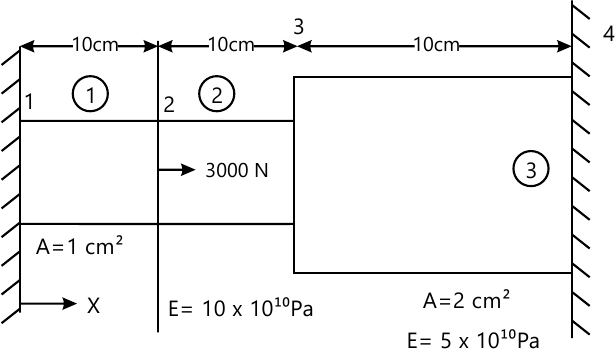(i)Determine the assembled (global) stiffness matrix, the displacement at nodes 2 and 3 and the reaction at nodes 1 and 4.
(ii) State the properties of the global stiffness matrix that you have developed.
(iii) How would you determine the size of the semi band width of the above stiffness matrix?Justify the statement that "the size of the semi band width of the stiffness matrix depends upon the numbering system adopted for nodes 'by actual calculation

14 M
1(b) Consider a linear bar element in one dimension with two nodes.
The fields variable is displacement u.Derive the shape function and the strain displacement relation matrix B.Also obtain the element stiffness matrix from the formula given by.$(k)^{e} =\int_{0}^{l}(B)^{T} (B)EA dx$
6 M

2(a) Give one parameter Galerkin solution of the following equation, for the domain shown in the figure 2
$-\left(\dfrac{\partial^{2}u}{\partial x^{2}}+\dfrac{\partial^{2}u}{\partial y^{2}}\right)=1$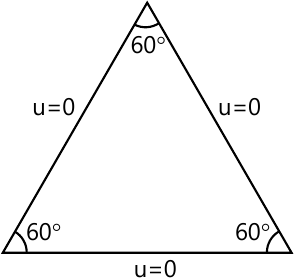10 M
2(b) Define aspect ratio.For the shapes of the element shown in figure 2 above,what will be the aspect ratio?What is the effect of aspect ratio on the accuracy of the FEA analysis?
5 M
2(c) Explain: Subparamatric, isoparametric and Superarametric elements.
5 M

3(a) For the plane truss shown in the figure 3
(i)Determine the displacement at nodes
(ii)Determine the stresses I each bar and
(iii)verify the nodal equilibrium at node 1.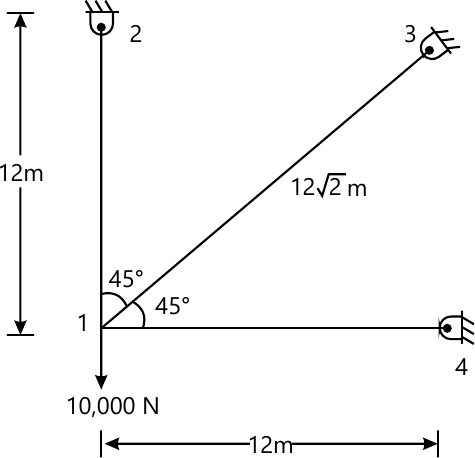For all bars:
E=30(10)7 pa
A=0.02 m2
15 M
3(b) Prove that in two noded bar element used for solving the problem of a rod to axial loads,the strains are expected to be more accurate at the midpoint of the element.
5 M

4(a) The governing differential equation for the steady state one dimensional conduction heat transfer with convection heat loss lateral surfaces is given by
$K\dfrac{d^{2}T}{dx^{2}}+q =\left(\dfrac{P}{A_{c}}h(T-T_{\infty})\right)$
Where
k = coefficient of thermal conductivity of the material
T=temperature
q =internal heat source per unit volume
P=perimeter
Ac =the cross-sectional area
H= Convective heat transfer coefficient and
T= ambient Temperature,
Develop the infinite element formulation for linear element. Use Rayleigh-Ritz method mapped over general element. Derive the relevant element level matrix equation.
14 M
4(b) Consider a two degree of freedom spring mas system as shown in th efigure 4.X1 and x2(t) are the independent generalized coordinates,determine the required equation of motion in matrix from.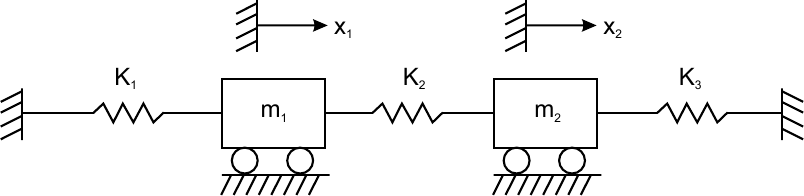6 M

5(a) Find the time dependent temperature a distribution in a plane wall that is insulated on one face and is subjected to a step change in surface temperature on the other face as shown in the figure 5 below using one finite element (Assume at t=0,T2=T0)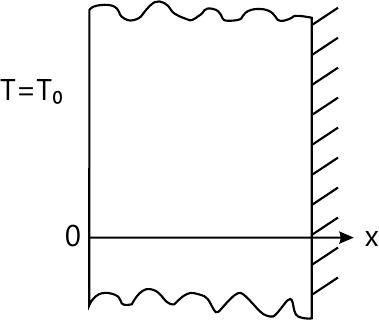10 M
5(b) Solve the following differential equation using method of Least square or point collocation Method (assume collection points x=0.25 & 0.5)
$\dfrac{d^{2}\phi}{dx^{2}}-\phi=x$ ,use boundary condition ϕ=(x=0) = 0 and ϕ(x=1)=0 compare your answer with exact solution at x=0.25,0.5 & 0.75.
10 M

6(a) A typical four-noded rectangular elements is shown in the figure.Derive the shape function for the temperature fields
If the nodes 1,2,3 and 4 are located at the coordinates point (3,3),(6,3), (6,5)& (3,5) respectively and the temperature distribution has been computed at each nodes as T1=120°C,T2=65°C ,T3 =40°C and T4=80°C.Derive the shape function and compute the temperature at x=4 and y=4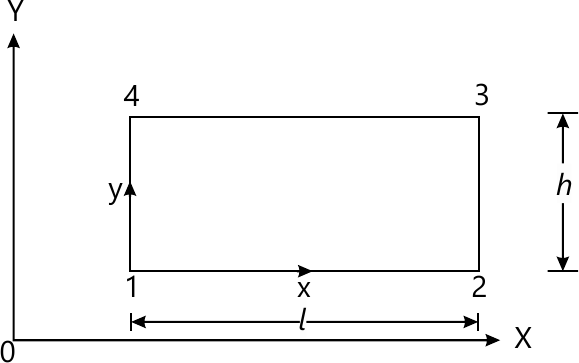12 M
6(b) Evaluate the integral $l = \int_{-1}^{1} (3^{x}-x)dx$
Newton cotes method Using 3 sampling points
 r W1 W2 W3 1 1 2 1/2 1/2 3 1/6 4/6 1/6

 r ϵ W1 1 0.00 2.00 2 ±0.57735 1.00 3 0.00 0.8888889 ± 0.7745966 0.5555555
8 M

7(a) For the triangular elements defined by nodes1: (5,5), 2(20,6) and 3:(6,30) obtain the strain displacement relation matrix B and determine the strain εx, εyxy. The displacement at the nodes are: U1=0.1, U2=0.4, U3=0.3 and V1=0.1, V2=0.5, V3=0.3
Assume the unit of displacements and the coordinates are the same. Also determine the displacements at point P(12,10)
10 M
Any two
7(b) Writes short notes on(any two)
Boundary Condition
Errors in FEA
Mesh refinement
Six noded triangular element
10 M

More question papers from Finite Element Analysis